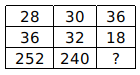Question 4

# Study the given pattern carefully and select the number that can replace the question mark (?) in it.Solution

(28$$\times 36)\div 4 = 1008/4 = 252$$
(30$$\times 32)\div 4 = 960/4 = 240$$
Similarly,
(36$$\times 18)\div 4 = 648/4 = 162$$
$$\therefore$$ The correct answer is option D.